# ML Aggarwal Class 6 Solutions for ICSE Maths Chapter 8 Ratio and Proportion Ex 8.1

## ML Aggarwal Class 6 Solutions for ICSE Maths Chapter 8 Ratio and Proportion Ex 8.1

Question 1.
Express the following ratios in simplest form:
(i) 20 : 40
(ii) 40 : 20
(iii) 81 : 108
(iv) 98 : 63
Solution: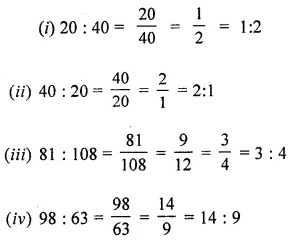Question 2.
Fill in the missing numbers in the following equivalent ratios: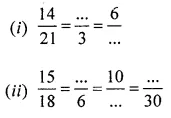Solution:Question 3.
Find the ratio of each of the following in simplest form :
(i) 2.1 m to 1.2 m
(ii) 91 cm to 1.04m
(iii) 3.5 kg to 250gm
(iv) 60 paise to 4 rupees
(v) 1 minute to 15 seconds
(vi) 15 mm to 2 cm
Solution: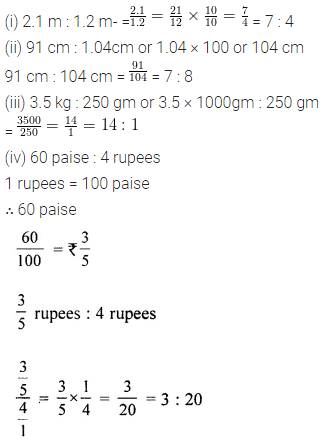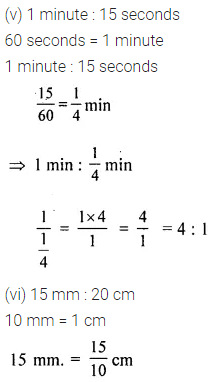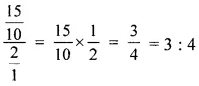Question 4.
The length and the breadth of a rectangular park are 125 m and 60 m respectively. What is the ratio of the length to the breadth of the park?
Solution:Question 5.
The population of village is 4800. If the numbers of females is 2160, find the ratio of males to that of females.
Solution:Question 6.
In a class, there are 30 boys and 25 girls. Find the ratio of the numbers of
(i) boys to that of girls.
(ii) girls to that of total number of students.
(iii) boys to that of total numbers of students.
Solution: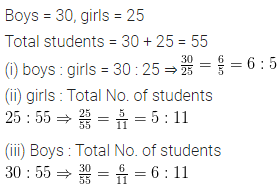Question 7.
In a year, Reena earns ₹ 1,50,000 and saves ₹ 50,000. Find the ratio of
(i) money she earns to the money she saves.
(ii) money that she saves to the money she spends.
Solution: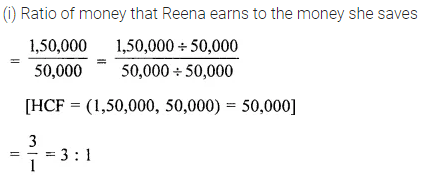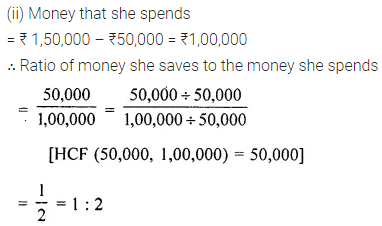Question 8.
The monthly expenses of a student have increased from ₹350 to ₹500. Find the ratio of
(i) increase in expenses and original expenses.
(ii) original expenses to increased expenses.
(iii) increased expenses to increased in expenses.
Solution: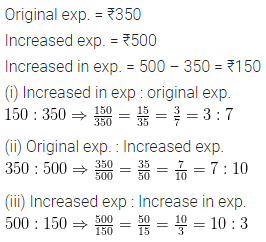Question 9.
Mr Mahajan and his wife are both school teachers and earn ₹20900 and ₹ 18700 per month respectively. Find the ratio of
(i) Mr Mahajan’s income to his wife’s income.
(ii) Mrs Mahajan’s income to the total income of both.
Solution: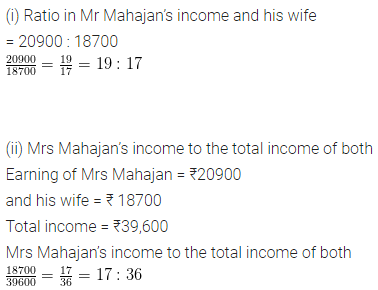Question 10.
Out of 30 students in a class, 6 like football, 12 like cricket and remaining like tennis. Find the ratio of
(a) Number of students liking football to number of students liking tennis.
(b) Number of students liking cricket to total number of students.
Solution:Question 11.
Divide ₹ 560 between Ramu and Munni in the ratio 3 : 2.
Solution: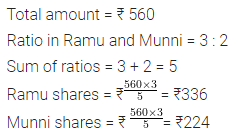Question 12.
Two people invested ₹ 15000 and ₹25000 respectively to start a business. They decided to share the profits in the ratio of their investments. If their profit is ₹ 12000, how much does each get?
Solution:Question 13.
The ratio of Ankur’s money to Roma’s money is 9 : 11. if Ankur has ₹540, how much money does Roma have?
Solution: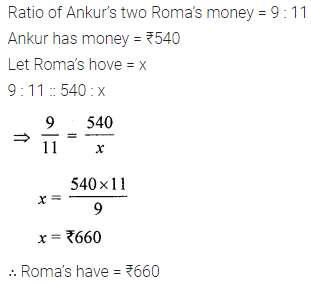Question 14.
The ratio of weights of tin and zinc in on alloy is 2 : 5. How much zinc is there in 31.5g of alloy?
Solution: